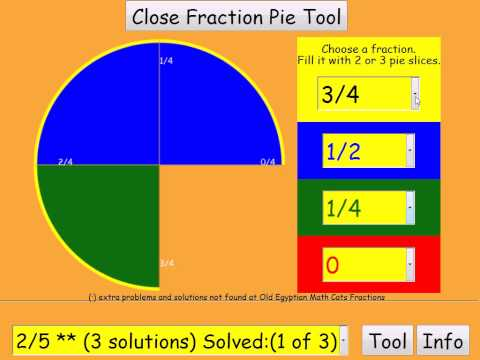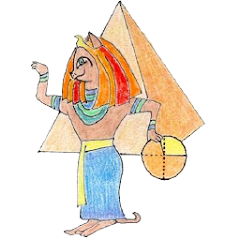# Old Egyptian Fractions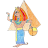1K+Everyone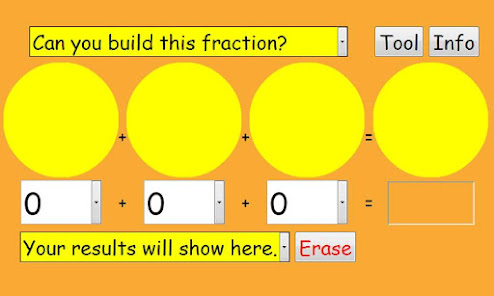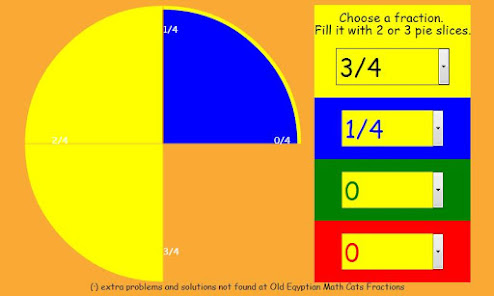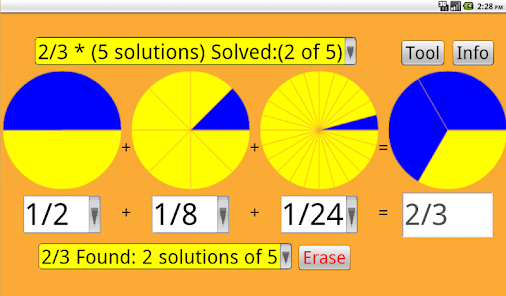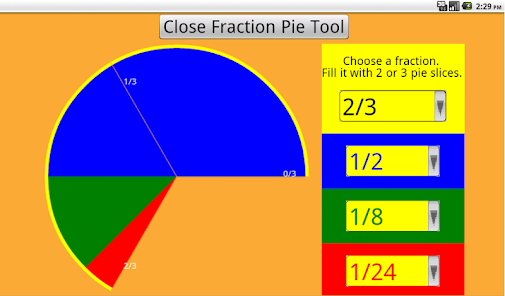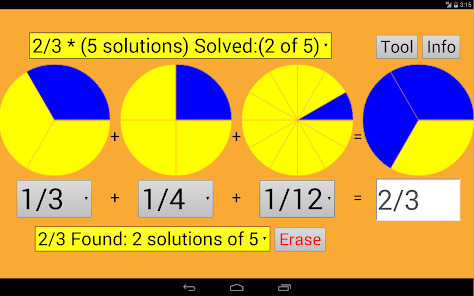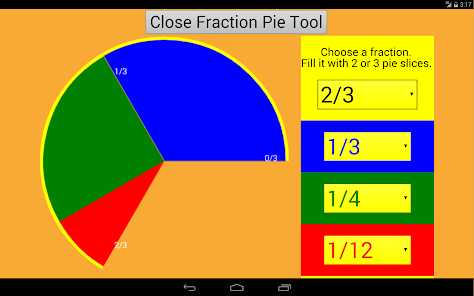This is the android version of the Mathcats Old Egyptian Fractions:
The app is about: proper fractions and their equivalent egyptian fractions (which in this case, are the addition of 2 or 3 unit fractions).

Old Egyptian Math Cats knew fractions like 1/2 or 1/4 (one piece of a pie).
But to make fractions like 3/4, they had to add pieces of pies like 1/2 + 1/4 = 3/4.
Old Egyptian Math cats never repeated the same fraction when adding.
They never wrote: 1/4 + 1/4 + 1/4 = 3/4

In ancient Egypt, they wrote fractions with numerator 1 or as a sum of fractions with numerator 1. So the fraction 2/3 would be 1/2 +1 / 6. It has a certain logic if we consider a particular case: if we divide two loaves among three people will give each bread half (1/2) and a third of the bread is left half (1/6).

How it works:

Choose puzzles from the list on the top. ( * = easier, **** = very hard.)
Add 2 or 3 fraction pieces below.
After you find one solution, the puzzle is marked "Solved."
Can you find more solutions? (Click to see them listed on the bottom.)

You can play with the internet version of Old Egyptian Fractions at:
http://www.mathcats.com/explore/oldegyptianfractions/index.html

Old Egyptians reference:
Rhind mathematical papyrus RMP: http://en.wikipedia.org/wiki/Rhind_Mathematical_Papyrus

Math Forum: Math Tools reference:
http://mathforum.org/mathtools/tool/233557/
Courses:
Math 1, 2, 3 - Fractions
Math 4, 5, 6, 7 - Writing fractions, Equivalent fractions, Comparing & ordering, Simplifying fractions, Adding fractions, Subtracting fractions, Multiplying fractions, Dividing fractions

Thanks for the reference:
http://srvcnpbs.xtec.cat/creamat/joomla/index.php/hem-vist/67/1250-aplicacio-per-a-android-sobre-fraccions-egipcies

This program is a work in collaboration:
Wendy Petti of Mathcats: http://www.mathcats.com
Maurici Carbó of Nummolt: http://www.nummolt.com
Boni Córdoba: Egyptian Cat drawing

MATHCATS APPS
Updated on
Oct 10, 2020

## Data safety

Safety starts with understanding how developers collect and share your data. Data privacy and security practices may vary based on your use, region, and age. The developer provided this information and may update it over time.No data shared with third parties